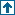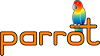parrotcode: Rational numbers PMC Contents | Dynamic PMCs

# NAMEsrc/dynpmc/rational.pmc - Rational numbers PMC

# DESCRIPTIONRational is currently going to get implemented. It uses the GNU Multiple Precision (GMP) library, like BigInt, because implementation with integers would be too unstable and inaccurate.

'LispRational' will subclass this.

You may use Rational with any of parrot's basic data types as well as with String-, Integer- and Float-PMCs.

# FunctionsCurrently `rational.pmc` only has some `static` functions for code sharing.

• static STRING *rat_get_string_to_base(PARROT_INTERP, PMC *self, int base)
• Returns a string representation of a Rational-PMC to a certain base.

• static void rat_add_integer(PARROT_INTERP, PMC *self, int value, PMC *dest)
• Adds an integer "value" to a Rational-PMC and stores the result in (another) Rational-PMC.

• static void rat_add_float(PARROT_INTERP, PMC *self, double value, PMC *dest)
• Adds a float "value" to a Rational-PMC and stores the result in (another) Rational-PMC. "value" is first conveted to a rational using GMPs mpq_set_d-function. This is meant to be exact.

• static void rat_multiply_integer(PARROT_INTERP, PMC *self, int value, PMC *dest)
• Multiplys a Rational-PMC with an integer "value" and stores the result in (another) Rational-PMC.

• static void rat_multiply_float(PARROT_INTERP, PMC *self, double value, PMC *dest)
• Multiplies a Rational-PMC with a float "value" and stores the result in (another) Rational-PMC.

• static void rat_divide_integer(PARROT_INTERP, PMC *self, int value, PMC *dest)
• Divides a Rational-PMC through an integer "value" and stores the result in (another) Rational-PMC.

• static void rat_divide_float(PARROT_INTERP, PMC *self, double value, PMC *dest)
• Divides a Rational-PMC through a float "value" and stores the result in (another) Rational-PMC.

• static void rat_power_int(PARROT_INTERP, PMC *self, int value, PMC *dest)
• Calculates the power of a Rational-PMC to an exponent value and stores the result in (another) Rational-PMC.

## MethodsThe DynPMC Rational has the following methods. Note, that all methods depend on GMP. If GMP is not available, an exception is thrown in almost all cases. The only exception is the version-method.

`METHOD version()`

Returns the version of GNU Multiple Precision library. Returns 0.0.0, if GMP is not available.

`void init()`

`void clone()`

`void destroy()`

`void set_integer_native(INTVAL)`

`void set_number_native(FLOATVAL)`

`void set_string_native(STRING*)`

`void set_string_keyed_int(INTVAL base, STRING *value)`

`INTVAL get_integer()`

`get_number()`

`get_bool()`

`STRING *get_string()`

`STRING *get_string_keyed_int(INTVAL base)`

`void increment()`

`void decrement()`

`PMC *add(PMC *value, PMC *dest)`

Adds Integer-, Float- or Rational-PMCs to SELF and stores them in dest.

`void i_add(PMC *value)`

`PMC *add_int(INTVAL value, PMC *dest)`

`void i_add_int(INTVAL value)`

`PMC *add_float(FLOATVAL value, PMC *dest)`

`void i_add_float(FLOATVAL value)`

`PMC *subtract(PMC *value, PMC *dest)`

`void i_subtract(PMC *value)`

`PMC *subtract_int(INTVAL value, PMC *dest)`

`void i_subtract_int(INTVAL value)`

`PMC *subtract_float(FLOATVAL value, PMC *dest)`

`void i_subtract_float(FLOATVAL value)`

`PMC *multiply(PMC *value, PMC *dest)`

`void i_multiply(PMC *value)`

`PMC *multiply_int(INTVAL value, PMC *dest)`

`void i_multiply_int(INTVAL value)`

`PMC *multiply_float(FLOATVAL value, PMC *dest)`

`void i_multiply_float(FLOATVAL value)`

`PMC *divide(PMC *value, PMC *dest)`

`void i_divide(PMC *value)`

`PMC *divide_int(INTVAL value, PMC *dest)`

`void i_divide_int(INTVAL value)`

`PMC *divide_float(FLOATVAL value, PMC *dest)`

`void i_divide_float(FLOATVAL value)`

`PMC *negate(PMC *dest)`

`void i_negate()`

`PMC *absolute(PMC *dest)`

`void i_absolute()`

`INTVAL cmp(PMC *value)`

`INTVAL is_equal(PMC *value)`